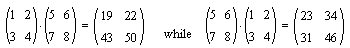# Multiplication of Matrices

Matrices are rectangular arrays with entries from an arbitrary field. An m × n (read "m by n") matrix is thus an array (aik) where i changes from 1 through m whereas k ranges from 1 through n. More explicitly,

a11 a12 a13 ... a1n
a21 a22 a23 ... a2n
a31 a32 a33 ... a3n
...
am1 am2 am3 ... amn

which has m rows and n columns.

With every m × n matrix A we may associate m row (1 × n) vectors ai, and n column (m × 1) vectors a,k. Should there be two matrices: an m×n matrix A = (aik) and an m×p matrix B = (bks), their product is defined as an m×p matrix C = (cis), where

cis = (ai,.b,s)

and inside parentheses I used the scalar (dot) product of two vectors.

The first thing to note is that not any two matrices can be multiplied. To carry out the multiplication we must have the column dimension of the left factor equal to the row dimension of the right factor. Nonetheless, wherever defined, the product is associative and distributive relative to the standard matrix addition. Matrix multiplication changes dimensions; so it's hard to talk about a unit element in general. A fruitful approach is to confine the study to square (m=n) matrices of the same dimension. So, let's for a while assume that all matrices below have dimension nxn. The benefit is immediate: any two such matrices can be multiplied. Moreover, the product is a matrix with the same dimension. This may be expressed by saying that

For a fixed n, the set of all n×n matrices is closed under matrix addition and multiplication.

With respect to addition, this set is an abelian group. Adding multiplication makes it a ring. The unit element is uniquely defined by E = (eik), where eik is the Kronecker's symbol eik = 1 iff i = k, and eik = 0, otherwise. (In matrix theory, the matrix is known as the identity matrix. All elements of an identity matrix are zero, except for the main diagonal, where all elements are 1.)

Not all square matrices are invertible. But, if both A and B are, then so is their product AB. Furthermore,

(AB)-1 = B-1A-1

This is verified formally:

(B-1A-1)(AB) = B-1(A-1A)B = B-1EB = B-1(EB) = B-1B = E.

and in a similar manner (AB)(B-1A-1) = E. However, in general, AB≠BA. For example,Thus the set of all invertible matrices does not form a field. To get a field we might restrict our discussion even further to the set of invertible diagonal matrices. A matrix A is diagonal if all its off-diagonal elements are zero: aik = 0 if i ≠ k. A diagonal matrix is invertible iff aii ≠ 0 for all i.To get a feeling for the definitions and facts claimed above, it's best to consider circumstances in which the theory acquires an intuitive meaning. In short, matrices give numerical expression to linear transformations of vector spaces.### What Can Be Multiplied?